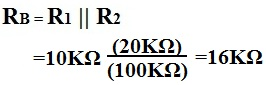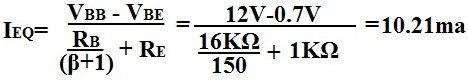﻿ Voltage Divider Bias of a BJT Transistor# Voltage Divider Bias of a BJT TransistorOne way to bias a BJT transistor is a method called voltage divider bias.

Voltage divider bias is the most popular and used way to bias a transistor. It uses a few resistors to make sure that voltage is divided and distributed into the transistor at correct levels. One resistor, the emitter resistor, RE also helps provide stability against variations in β that may exist from transistor to transistor.

Below is a typical BJT receiving voltage divider bias:For the circuit above, we're going to assume that β=100 for the transistor.

The base supply voltage, VBB, is calculated by:We calculate RB below, which we will use the next calculation for IE.Then, we calculate for the emitter current using the following formula:The collector current IC is approximately equal to the emitter current.

IC≈ IE

### How Emitter Resistor, RE, Fights Against the Instability of β

The RE provides stability in gain of the emitter current of a transistor circuit. of a transistor, its gain or amplification factor, can vary by large amounts from transistor to transistor, even if they're the same exact type from the same batch. There is no way to replicate the same exact βs across transistors. Therefore, when we are designing transistor circuits where we want roughly the same gain in all of them, we must design them in a way that produces the same gain despite fluctuations in the β values. We do this by carefully choosing the emitter resistance, RE, which provides stability against differences in β. RE provides stability in gain of the output current of a transistor circuit.For the above transistor, the β was equal to 100. But let's say we have to swamp out that transistor and the new transistor has a β of 150. What will the new calculation be, and will the output current of the transistor swing largely in changes to the β or will it vary little and still be stable?The answer is, the transistor output current doesn't vary much despite the large difference in β values of the transistors. Even though there is a difference of 50 of β in the transistors, there is less than a half of milliampere difference in the output current, IE. If wanted, an even larger value of RE can be used so that there is even more stability against the β of transistors. However, realize that the larger RE is, the more gain that is lost for amplification in the circuit. So there must be balance in the design of the value of RE.

### Advantages of the Voltage Divider Bias

Again, voltage divider bias is the most popular and used way to bias BJT transistors. The resistors help to give complete control over the voltage and current that each region receives in the transistor. And the emitter resistor, RE, allows for stability of the gain of the transistor, despite fluctations in the β values.